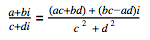IMDIV

Returns one complex number divided by another.

Sample Usage

IMDIV("11+16i", "3+2i")

IMDIV("4+2j", 2)

Syntax

IMDIV(dividend, divisor)

• dividend - The complex number to be divided.
• divisor - The complex number to divide by.

Notes

• The division between two complex numbers is defined as follows:
•• You can divide two complex numbers only if they have the same suffix (i or j). For example, you can't do IMDIV("4+3i", "1+2j").

• COMPLEX: The COMPLEX function creates a complex number, given real and imaginary coefficients.
• IMREAL: Returns the real coefficient of a complex number.
• IMAGINARY: Returns the imaginary coefficient of a complex number.
• IMPRODUCT: Returns the result of multiplying a series of complex numbers together.

Examples

1 A B
2 Formula Result
3 =IMDIV("11+16i", "3+2i") 5+2i
4 =IMDIV("4+2j", 2) 2+j
5 =IMDIV(COMPLEX(11, 16), COMPLEX(3, 2)) 5+2i